Related Articles

# Class 10 NCERT Solutions- Chapter 13 Surface Areas And Volumes – Exercise 13.4

• Difficulty Level : Medium
• Last Updated : 04 Mar, 2021

### Question 1. A drinking glass is in the shape of a frustum of a cone of height 14 cm. The diameters of its two circular ends are 4 cm and 2 cm. Find the capacity of the glass.

Solution:

Given values:

Height of frustum (h) = 14 cm

Radius of larger circle end (R) == 2 cm

Radius of smaller circle end (r)== 1 cm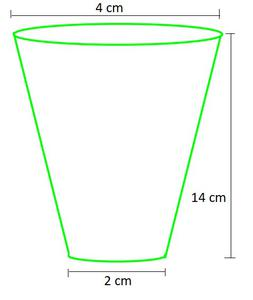Capacity of frustum-shaped glass = Volume of Frustumπh (r2 + R2 + rR)× π × 14 ((1 × 1) + (2 × 2) × (2 × 1))× 14 × 7                     (taking π=)= 102.67 cm3

Hence, the capacity of frustum-shaped glass = 102.67 cm3

### Question 2. The slant height of a frustum of a cone is 4 cm and the perimeters (circumference) of its circular ends are 18 cm and 6 cm. Find the curved surface area of the frustum.

Solution:

Slant height of frustum (l) = 4 cm

Let radius of smaller circle end = r

Let radius of larger circle end = R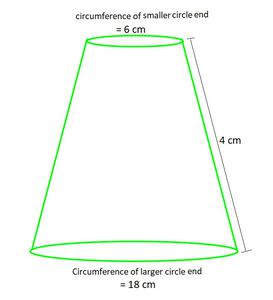Circumference of circle = 2π × (radius of circle)

Circumference of larger circle = 2πR

18 cm2 = 2πR

R =R =cm

Circumference of smaller circle = 2πr

6 cm2 = 2πr

r =r =cm

Now, as curve surface area of frustum = π (r+R) l

= π × () × 4

= 12 × 4                         (Taking π common and canceling it)

= 48cm2

Hence, the curved surface area of the frustum = 48cm2

### Question 3. A fez, the cap used by the Turks, is shaped like the frustum of a cone (see Fig.). If its radius on the open side is 10 cm, radius at the upper base is 4 cm and its slant height is 15 cm, find the area of material used for making it.

Solution:

Given values:

Slant height of frustum (l)= 15 cm

Let radius of smaller circle end (r) = 4 cm

Let radius of larger circle end (R) = 10 cm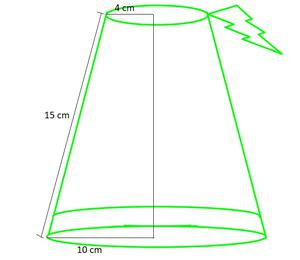Area of material used for making it = Curve surface area + area of upper base

= (π(r+R)l) + (πr2)

= π ((r+R)l + r2)                                              (Taking π common)

= π ((4+10) × 15 + (4 × 4))× (226)                                         (Taking π =)

= 710.286 cm2

Hence, the area of material used for making it = 710.286 cm

### Question 4. A container, opened from the top and made up of a metal sheet, is in the form of a frustum of a cone of height 16 cm with radii of its lower and upper ends as 8 cm and 20 cm, respectively. Find the cost of the milk which can completely fill the container, at the rate of ₹ 20 per litre. Also find the cost of metal sheet used to make the container, if it costs ₹ 8 per 100 cm2. (Take π = 3.14)

Solution:

Given values:

Height of frustum (h)= 16 cm

Let radius of smaller circle end (r) = 8 cm

Let radius of larger circle end (R) = 20 cm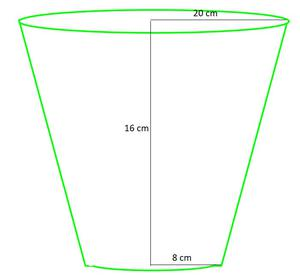The amount of milk to fill the container = Volume of frustumπh (r2 + R2 + rR)× 3.14 × 16 (8×8 + 20×20 + 8×20)                (Taking π=3.14)× 3.14 × 16 × (624)

= 10449.92 cm3

Cost of 1 litre milk = ₹ 20

And as, 1 m3 = 1000 cm3 = 1 litre

10449.92 cm3 = () ×10449.92 litres

cost of 10449.92 cm3 = () × 20

= ₹ 208.998

Now, metal sheet used to make the container = Curve surface area + area of lower base

= (π(r+R)l) + (πr2)

= π ((r+R)l + r2)                                              (Taking π common)

= π ((20+8) × (√(162+(20-8)2)) + (8 × 8))                                (Slant height (l) = √(h2+(R-r)2))

= 3.14 × (28 × √400 + 64)                                       (Taking π = 3.14)

= 3.14 × (624)

= 1959.36 cm2

Hence, the metal sheet used to make the container = 1959.36 cm2

As, cost of 100 cm2= ₹ 8

1959.36 cm2 = (8/100) × 1959.36

= ₹156.748

Hence, the cost of the milk which can completely fill the container = ₹ 208.998

and, the cost of metal sheet used to make the container = ₹156.748

### Question 5. A metallic right circular cone 20 cm high and whose vertical angle is 60° is cut into two parts at the middle of its height by a plane parallel to its base. If the frustum so obtained be drawn into a wire of diameter 1/16 cm, find the length of the wire.

Solution:

As the angle is cut into two equal parts, the height gets half too.

Let radius of smaller circle end = r

Let radius of larger circle end = R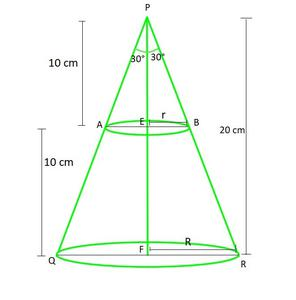In ∆PFR and ∆PEB

tan ∝ =tan 30° =R =r =and as height of frustum = 10 cm

So according to the question,

Frustum is converted to cylindrical wire having diametercm

Volume of Frustum = Volume of Cylinder

Volume of Frustum =πh (r2 + R2 + rR)cm3  ………………………..(1)

Volume of Cylinder =π(radius)2Hπ()2H …………………(2)

As (1) = (2) , then

7000π / 9 = 1/3 π(1/(16×2))2H

H =(cancel π from both side)

H = 796444.443 cm

H = 7964.44 m

Hence, the length of the wire = 7964.44 m

Attention reader! All those who say programming isn’t for kids, just haven’t met the right mentors yet. Join the  Demo Class for First Step to Coding Coursespecifically designed for students of class 8 to 12.

The students will get to learn more about the world of programming in these free classes which will definitely help them in making a wise career choice in the future.

My Personal Notes arrow_drop_up